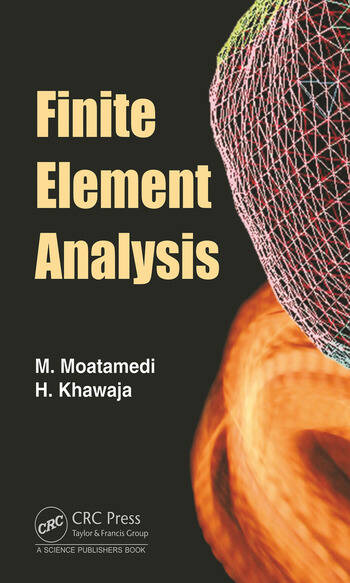# Finite Element Analysis

## 1st Edition

M Moatamedi, Hassan A. Khawaja

CRC Press
Published July 27, 2018
Reference - 154 Pages - 59 B/W Illustrations
ISBN 9781138320734 - CAT# K391767

was \$179.95

USD\$143.96

SAVE ~\$35.99

FREE Standard Shipping!

## Preview

### Summary

Finite element analysis has become the most popular technique for studying engineering structures in detail. It is particularly useful whenever the complexity of the geometry or of the loading is such that alternative methods are inappropriate. The finite element method is based on the premise that a complex structure can be broken down into finitely many smaller pieces (elements), the behaviour of each of which is known or can be postulated. These elements might then be assembled in some sense to model the behaviour of the structure. Intuitively this premise seems reasonable, but there are many important questions that need to be answered. In order to answer them it is necessary to apply a degree of mathematical rigour to the development of finite element techniques. The approach that will be taken in this book is to develop the fundamental ideas and methodologies based on an intuitive engineering approach, and then to support them with appropriate mathematical proofs where necessary. It will rapidly become clear that the finite element method is an extremely powerful tool for the analysis of structures (and for other field problems), but that the volume of calculations required to solve all but the most trivial of them is such that the assistance of a computer is necessary.

As stated above, many questions arise concerning finite element analysis. Some of these questions are associated with the fundamental mathematical formulations, some with numerical solution techniques, and others with the practical application of the method. In order to answer these questions, the engineer/analyst needs to understand both the nature and limitations of the finite element approximation and the fundamental behaviour of the structure. Misapplication of finite element analysis programs is most likely to arise when the analyst is ignorant of engineering phenomena.